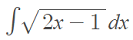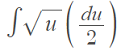# U Substitution (Reverse Chain Rule)

Integrals > U Substitution

Contents:

1. Overview and Basic Example
2. U Substitution for:

## 1. Overview and Basic Example

U substitution (also called integration by substitution or u substitution) takes a rather complicated integral and uses a change of variable to make the integration simpler.

U substitution requires strong algebra skills and knowledge of rules of differentiation. Why? Because you’ll need to be able to look at the integral and see where a little algebra might get the problem into one you can easily integrate. Integration is really reverse-differentiation, so knowing your rules of differentiation will make the task much easier.

## Example Problem

Example problem #1: Integrate the following using integration by substitution.:Step 1: Choose a term to substitute for u. You want to make the expression look as simple as possible. If you’re not sure, replace the inside function, which works most of the time.

For this example, I’ve chosen 2x – 1, the inside function, for u.

Step 2: Calculate the differential. We have changed “x” to “u” for the inside function, so we need to carry this logic through to “du”. To do this, differentiate the function you chose in Step 1, using the rule:

If u = g(x), then du = g′(x) dx

u = 2x + 1, so du = 2 dx → dx = du/2.

Rewriting the integral with our two replacements u = 2x + 1 and dx = 2 du, we have:Step 3: Bring any constants out in front (because of the constant multiple rule for integrals):Step 4: Integrate in terms of u:Step 5: Simplify:Step 6: Replace u with your original function. In other words, reverse the substitution you made in Step 1:## U Substitution Trigonometric Functions: Examples

Example problem #1: Integrate ∫sin 3x dx.

Step 1: Select a term for “u.” Look for substitution that will result in a more familiar equation to integrate. Substituting u for 3x will leave an easier term to integrate (sin u), so:

• u = 3x

Step 2: Differentiate u:

• du = 3 dx

Or (rewriting using algebra—necessary because you need to replace “dx”, not 3 dx):
⅓ du = dx

Step 3: Replace all forms of x in the original equation:

• Substituting for u: ∫ sin 3x dx = ∫ sin u dx
• Substituting for dx: ∫ sin u dx = ∫ sin u ⅓ du

Step 4: Rewrite, bringing the constant in front of the integral symbol (so that you can easily integrate):

• ∫ sin u ⅓ du = ⅓ ∫ sin u du

Step 5: Integrate using the usual rules of integration:

• ⅓ ∫ sin u du = ⅓ (-cos u) + C = -⅓ cos u + C

Step 6: Re-substitute for u:
“u” is left in the equation, so:

• ⅓ cos u + C = ⅓ cos 3x + C

That’s it!

Example problem #2: Integrate ∫ 5 sec 4x dx

Step 1: Pick a term to substitute for u:

• u = 4x

Step 2: Differentiate, using the usual rules of differentiation.

• du = 4 dx
• ¼ du = dx (using algebra to rewrite, as you need to substitute dx on its own, not 4x)

Step 3: Substitute u and du into the equation:

• ∫ 5 sec 4x tan 4x dx = 5 ∫ sec u tan u ¼ du =
• 54 ∫ sec u tan u du

Step 4: Integrate, using the usual rules of integration. For this problem, integrate using the rule D(sec x) = sec x tan x:

• 54∫ sec u tan u du = 54 sec u + C

Step 5: Re-substitute for u:

• 54 sec u + C = 54 sec 4x + C

Tip: If you don’t know the rules by heart, compare your function to the general rules of integration and look for familiar looking integrands before you attempt to substitute anything for u.

That’s all there is to U Substitution for Trigonometric Functions!

## U Substitution for Definite Integrals

In general, a definite integral is a good candidate for u substitution if the equation contains both a function and that function’s derivative. When evaluating definite integrals, figure out the indefinite integral first and then evaluate for the given limits of integration.

Example problem: Evaluate:Step 1: Pick a term for u. Choose sin x for this example problem, because the derivative is cos x.
u = sin x.

Step 2: Find the derivative of u:

• du = cos x dx

## 4. U Substitution for Exponential Functions

Example question: Find the integral for the exponential function, using u substitution.Step 1: Rewrite your function using algebra to get it in a form where you can easily find an integral:Step 2: Split the function into separate parts:

• ∫(1 + e – x)dx =
• ∫1dx + ∫e – xdx

Step 3: Pick u and find the derivative of u. For this example, pick “-x” in e-x:

• u = -x
• du = -1·dx

Step 4: Find a way to remove the symbol dx using your second substitution in Step 3. Using algebra:

• du =-1·dx, so
• -1du = dx

Step 5: Substitute the “u”, and “du” from Steps 3 and 4 into the equation.

• ∫1dx + ∫eu(-1)du

Step 6: Solve the integrals:

• ∫1dx + ∫eu(-1)du = x – eu + C

Step 7: Re-substitute your terms back into the function. u = -x, so:

• x – eu + C = x – e-x + C

That’s it!

CITE THIS AS:
Stephanie Glen. "U Substitution (Reverse Chain Rule)" From StatisticsHowTo.com: Elementary Statistics for the rest of us! https://www.statisticshowto.com/u-substitution/